### Home > INT2 > Chapter 3 > Lesson 3.1.3 > Problem3-34

3-34.

Examine the diagrams below. For each one, write and solve an equation to calculate the value of $x$. Show all work.

1.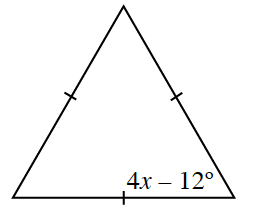Equilateral triangles have three congruent angles.

1.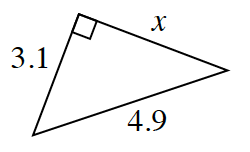This is a right triangle, so use the Pythagorean Theorem to solve for $x$.

1.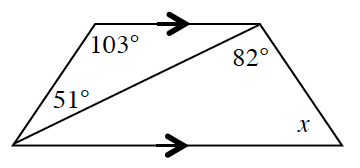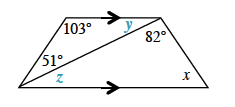$∠y = 180^\circ − 103^\circ − 51^\circ$
$∠y = 26^\circ$

Because $∠y$ and $∠z$ are alternate interior angles,
they are congruent. Therefore, $x = 180^\circ − 82^\circ − 26^\circ$.

$x = 72^\circ$

1.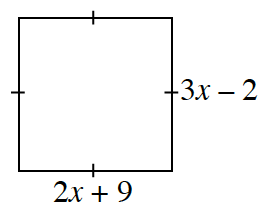$3x - 2 = 2x + 9$